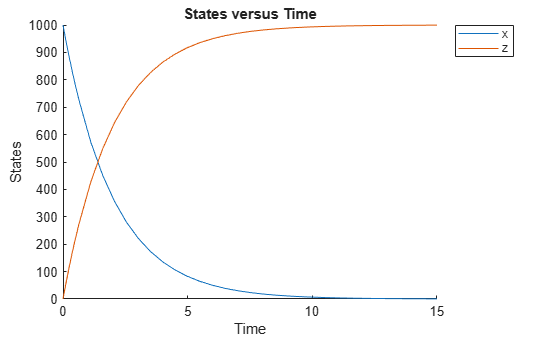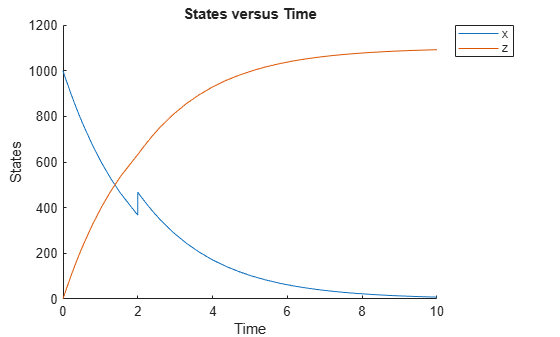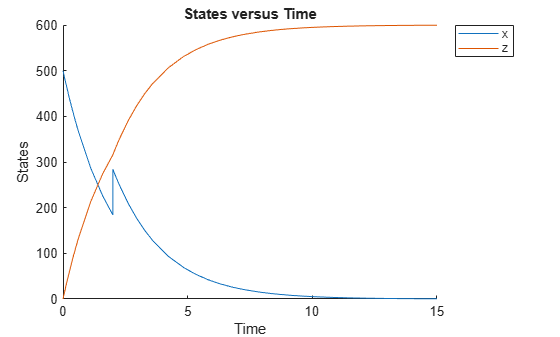# verify (model, variant)

Validate and verify SimBiology model

## Syntax

``verify(modelObj)``
``verify(modelObj,csObj)``
``verify(modelObj,dvObj)``
``verify(modelObj,csObj,dvObj)``
``verify(modelObj,csObj,variantObj,doseObj)``

## Description

example

````verify(modelObj)` performs checks on a `Model` `modelObj` to verify that you can simulate the model. This function generates stacked errors and warnings if it finds any problems. To see the entire list of errors and warnings, use `sbiolasterror` and `sbiolastwarning`. The function uses the active configuration set, any active doses and active variants for verification.```

example

````verify(modelObj,csObj)` verifies a model `modelObj` using the specified configset object `csObj` and any active variants and active doses. Any other configsets are ignored. If you set `csObj` to empty `[]`, the function uses the active configset.```

example

````verify(modelObj,dvObj)` verifies a model `modelObj` using doses or variants specified by `dvObj` and the active configset. `dvObj` can be one of the following: ```Variant object``````ScheduleDose object````RepeatDose object` Array of doses or variants If you set `dvObj` to empty `[]`, the function uses the active configset, active variants, and active doses.If you specify `dvObj` as variants, the function uses the specified variants and active doses. Any other variants are ignored.If you specify `dvObj` as doses, the function uses the specified doses and active variants. Any other doses are ignored.```

example

````verify(modelObj,csObj,dvObj)` verifies a model `modelObj` using a configset object `csObj` and doses or variants specified by `dvObj`. If you set `csObj` to `[]`, then the function uses the active configset object. If you set `dvObj` to `[]`, then the function uses no variants, but uses active doses.If you specify `dvObj` as variants, the function uses the specified variants and active doses. Any other variants are ignored.If you specify `dvObj` as doses, the function uses the specified doses and active variants. Any other doses are ignored.```

example

````verify(modelObj,csObj,variantObj,doseObj)` verifies a model `modelObj` using a configset object `csObj`, variants (`variantObj`) and doses (`doseObj`). Any other configset, doses, and variants are ignored. If you set `csObj` to `[]`, then the function uses the active configset object. If you set `variantObj` to `[]`, then the function uses no variants. If you set `doseObj` to `[]`, then the function uses no doses.```

## Input Arguments

collapse all

SimBiology model, specified as a SimBiology model object.

Configuration set object, specified as a `Configset object` that stores simulation-specific information.

Dose or variant object, specified as a `ScheduleDose object` , `RepeatDose object` , an array of dose objects, `Variant object` , or an array of variant objects.

• When `dvObj` is a dose object, `verify` uses the specified dose object as well as any active variant objects if available.

• When `dvObj` is a variant object, `verify` uses the specified variant object as well as any active dose objects if available.

Variant object, specified as a `Variant object` or an array of variant objects.

Dose object, specified as a ```ScheduleDose object``` , `RepeatDose object` , or an array of dose objects. A dose object defines additions that are made to species amounts or parameter values.

## Examples

collapse all

`sbioloadproject radiodecay.sbproj`

Add a new configuration set using a different stop time of 15 seconds.

```csObj = addconfigset(m1,'newStopTimeConfigSet'); csObj.StopTime = 15;```

Verify the model while using the configset object.

`verify(m1,csObj);`

After verification, check the latest errors and warnings if there is any.

`sbiolasterror`
```ans = 0x1 empty struct array with fields: Type MessageID Message ```
`sbiolastwarning`
```ans = struct with fields: Type: 'Warning' MessageID: 'SimBiology:DimAnalysisNotDone_MatlabFcn_Dimensionless' Message: 'Cannot perform dimensional analysis for rule 'kempt definition' because of the function 'tanh' in the rule. Because UnitConversion is on, correct simulation results will depend on this expression being dimensionally correct. Additionally, SimBiology simulates the model in a unit system determined at runtime. The units are determined by the units used in the model and the model's configset. Unless the inputs and outputs to the function are dimensionless, results may change due to configset option changes, changes to the model, or version changes in SimBiology. It is recommended that input and output arguments to functions be dimensionless to ensure correct results....' ```

Simulate the model.

```sim = sbiosimulate(m1,csObj); sbioplot(sim);````sbioloadproject radiodecay.sbproj`

Get the default configuration set from the model.

`defaultConfigSet = getconfigset(m1,'default');`

Add a scheduled dose of 100 molecules at 2 seconds for species `x`.

```dObj = adddose(m1,'d1','schedule'); dObj.Amount = 100; dObj.AmountUnits = 'molecule'; dObj.TimeUnits = 'second'; dObj.Time = 2; dObj.TargetName = 'unnamed.x';```

Verify the model while using the default configset object and added dose object.

`verify(m1,defaultConfigSet,dObj);`

After verification, check the latest errors and warnings if there is any.

`sbiolasterror`
```ans = 0x1 empty struct array with fields: Type MessageID Message ```
`sbiolastwarning`
```ans = struct with fields: Type: 'Warning' MessageID: 'SimBiology:DimAnalysisNotDone_MatlabFcn_Dimensionless' Message: 'Cannot perform dimensional analysis for rule 'kempt definition' because of the function 'tanh' in the rule. Because UnitConversion is on, correct simulation results will depend on this expression being dimensionally correct. Additionally, SimBiology simulates the model in a unit system determined at runtime. The units are determined by the units used in the model and the model's configset. Unless the inputs and outputs to the function are dimensionless, results may change due to configset option changes, changes to the model, or version changes in SimBiology. It is recommended that input and output arguments to functions be dimensionless to ensure correct results....' ```

Simulate the model using the same configset and dose objects.

`sim = sbiosimulate(m1,defaultConfigSet,dObj);`

Plot the result.

`sbioplot(sim);``sbioloadproject radiodecay.sbproj`

Add a new configuration set using a different stop time of 15 seconds.

```csObj = m1.addconfigset('newStopTimeConfigSet'); csObj.StopTime = 15;```

Add a scheduled dose of 100 molecules at 2 seconds for species `x`.

```dObj = adddose(m1,'d1','schedule'); dObj.Amount = 100; dObj.AmountUnits = 'molecule'; dObj.TimeUnits = 'second'; dObj.Time = 2; dObj.TargetName = 'unnamed.x';```

Add a variant of species `x` using a different initial amount of 500 molecules.

```vObj = addvariant(m1,'v1'); addcontent(vObj,{'species','x','InitialAmount',500});```

Verify the model while using the configset, dose, and variant objects. Note that the order of arguments should be as described.

`verify(m1,csObj,vObj,dObj);`

After verification, check the latest errors and warnings if there is any.

`sbiolasterror`
```ans = 0x1 empty struct array with fields: Type MessageID Message ```
`sbiolastwarning`
```ans = struct with fields: Type: 'Warning' MessageID: 'SimBiology:DimAnalysisNotDone_MatlabFcn_Dimensionless' Message: 'Cannot perform dimensional analysis for rule 'kempt definition' because of the function 'tanh' in the rule. Because UnitConversion is on, correct simulation results will depend on this expression being dimensionally correct. Additionally, SimBiology simulates the model in a unit system determined at runtime. The units are determined by the units used in the model and the model's configset. Unless the inputs and outputs to the function are dimensionless, results may change due to configset option changes, changes to the model, or version changes in SimBiology. It is recommended that input and output arguments to functions be dimensionless to ensure correct results....' ```

Simulate the model using the same configset, variant, and dose objects.

`sim = sbiosimulate(m1,csObj,vObj,dObj);`

Plot the result.

`sbioplot(sim);`